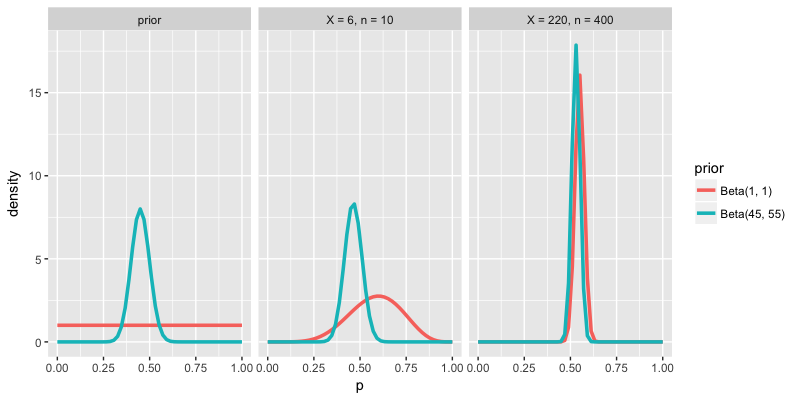# Influence of the prior & data on the posterior

Examine the density plots below. The first set of density plots illustrates 2 potential priors for your underlying support $$p$$. The other 2 sets of density plots illustrate the posterior models for $$p$$ that evolve from these different priors and different polling data $$X$$ and $$n$$. Which of the following best describes the combined influence of the prior and data on the posterior?